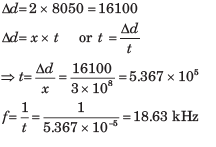Courses

# Spread Spectrum - MCQ Test

## 20 Questions MCQ Test GATE ECE (Electronics) 2022 Mock Test Series | Spread Spectrum - MCQ Test

Description
This mock test of Spread Spectrum - MCQ Test for Electronics and Communication Engineering (ECE) helps you for every Electronics and Communication Engineering (ECE) entrance exam. This contains 20 Multiple Choice Questions for Electronics and Communication Engineering (ECE) Spread Spectrum - MCQ Test (mcq) to study with solutions a complete question bank. The solved questions answers in this Spread Spectrum - MCQ Test quiz give you a good mix of easy questions and tough questions. Electronics and Communication Engineering (ECE) students definitely take this Spread Spectrum - MCQ Test exercise for a better result in the exam. You can find other Spread Spectrum - MCQ Test extra questions, long questions & short questions for Electronics and Communication Engineering (ECE) on EduRev as well by searching above.
QUESTION: 1

### A rate 1/2 convolution code with dfrec = 10 is used to encode a data requeence occurring at a rate of 1 kbps. The modulation is binary PSK. The DS spread spectrum sequence has a chip rate of 10 MHz Que: The coding gain is

Solution: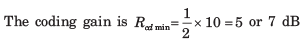QUESTION: 2

### A rate 1/2 convolution code with dfrec = 10 is used to encode a data requeence occurring at a rate of 1 kbps. The modulation is binary PSK. The DS spread spectrum sequence has a chip rate of 10 MHz Que: The processing gain is

Solution:

The processing gain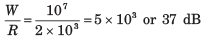QUESTION: 3

### A CDMA system is designed based on DS spread spectrum with a processing gain of 1000 and BPSK modulation scheme. If user has equal power and the desired level of performance of an error probability of 10-6, the number of user will be

Solution:

To achieve an error probability of 10-6 ,we required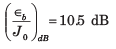Then, the number of users of the CDMA system is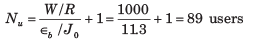QUESTION: 4

In previous question if processing gain is changed to 500, then number of users will be

Solution:

If the processing gain is reduced to W/R = 500, then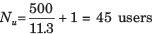QUESTION: 5

A DS spread spectrum system transmit at a rate of 1 kbps in the presets of a tone jammer. The jammer power is 20 dB greater then the desired signal, and the required εb/J0 to achieve satisfactory performance is 10 dB.

Que: The spreading bandwidth required to meet the specifications is

Solution:

We have a system where  (Jav/Pav) = 20 dB, R =1000, and (εb/J0)db = 10 dB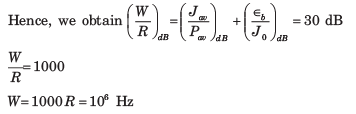QUESTION: 6

A DS spread spectrum system transmit at a rate of 1 kbps in the presets of a tone jammer. The jammer power is 20 dB greater then the desired signal, and the required εb/J0 to achieve satisfactory performance is 10 dB.

Que: If the jammer is a pulse jammer, then pulse duty cycle that results in worst case jamming is

Solution:

The duty cycle of a pulse jammer of worst-case jamming is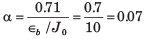QUESTION: 7

A DS spread spectrum system transmit at a rate of 1 kbps in the presets of a tone jammer. The jammer power is 20 dB greater then the desired signal, and the required εb/J0 to achieve satisfactory performance is 10 dB.

Que: The correspond probability of error is

Solution:

The corresponding probability of error for this worst-case jamming is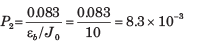QUESTION: 8

A CDMA system consist of 15 equal power user that transmit information at a rate of 10 kbps, each using a DS spread spectrum signal operating at chip rate of 1 MHz. The modulation scheme is BPSK

Que: The Processing gain is

Solution: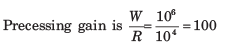QUESTION: 9

A CDMA system consist of 15 equal power user that transmit information at a rate of 10 kbps, each using a DS spread spectrum signal operating at chip rate of 1 MHz. The modulation scheme is BPSK

Que: The value of εb/Jb is

Solution:

We have N= 15 users transmitting at a rate of 10,000 bps each, in a bandwidth of W =1MHz.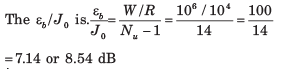QUESTION: 10

A CDMA system consist of 15 equal power user that transmit information at a rate of 10 kbps, each using a DS spread spectrum signal operating at chip rate of 1 MHz. The modulation scheme is BPSK

Que: How much should the processing gain be increased to allow for doubling the number of users with affecting the autopad SNR

Solution: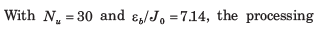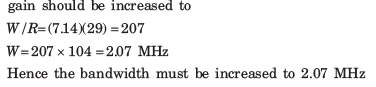QUESTION: 11

An m =10 ML shift register is used to generate the pre hdarandlm sequence in a DS spread spectrum system. The chip duration is Tc = ℓμs  and the bit duration is Tb, = NTc, where N is the length (period of the m sequence).

Que: The processing gain of the system is

Solution:

The period of the maximum length shift register sequence is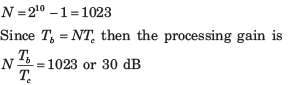QUESTION: 12

An m =10 ML shift register is used to generate the pre hdarandlm sequence in a DS spread spectrum system. The chip duration is Tc = ℓμs  and the bit duration is Tb, = NTc, where N is the length (period of the m sequence).

Que: If the required ε/J0 is 10 and the jammer is a tone jammer with an average power Jav, then jamming margin is.

Solution:

A Jamming margin is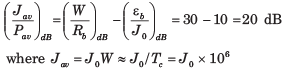QUESTION: 13

An FH binary orthogonal FSK system employs an m = 15 stage liner feedback shift register that generates an ML sequence. Each state of the shift register selects one of L non over lapping frequency bands in the hopping pattern. The bit rate is 100 bits/s. The demodulator employ non coherent detection.

Que: If the hop rate is one per bit, the hopping band width for this channel is

Solution:

The length of the shift-register sequence is

L = 2m - 1215 - 1 = 32767 bits

For binary FSK modulation, the minimum frequency separation is 2/T, where 1/T is the symbol (bit) rate.
The hop rate is 100 hops/sec. Since the shift register has L = 32767 states and each state utilizes a bandwidth of 2/T = 200 Hz, then the total bandwidth for the FH signal is 6.5534 MHz.

QUESTION: 14

An FH binary orthogonal FSK system employs an m = 15 stage liner feedback shift register that generates an ML sequence. Each state of the shift register selects one of L non over lapping frequency bands in the hopping pattern. The bit rate is 100 bits/s. The demodulator employ non coherent detection

Que: Suppose the hop rate is increased to 2 hops/bit and the receiver uses square law combining the signal over two hops. The hopping bandwidth for this channel is

Solution:

If the hopping rate is 2 hops/bit and the bit rate is 100 bits/sec, then, the hop rate is 200 hops/sec. The minimum frequency separation for orthogonality 2/T =400 Hz. Since there are N = 2767 states of the shift register and for each state we select one of two frequencies separated by 400 Hz, the hopping bandwidth is 13.1068 MHz.

QUESTION: 15

In a fast FH spread spectrum system, the information is transmitted via FSK with non coherent detection. Suppose there are N = 3 hops/bit with hard decision decoding of the signal in each hop. The channel is AWGN with power spectral density 1/2 N0 and an SNR 20-13 dB (total SNR over the three hops)

Que: The probability of error for this system is

Solution:

The total SNR for three hops is 20 ~ 13 dB. Therefore the SNR per hop is 20/3. The probability of a chip error with non-coherent detection is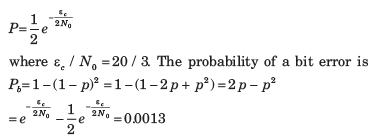QUESTION: 16

In a fast FH spread spectrum system, the information is transmitted via FSK with non coherent detection. Suppose there are N = 3 hops/bit with hard decision decoding of the signal in each hop. The channel is AWGN with power spectral density 1/2 N0 and an SNR 20-13 dB (total SNR over the three hops)

Que: In case of one hop per bit the probability of error is

Solution:

In the case of one hop per bit, the SNR per bit is 20,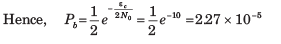QUESTION: 17

A slow FH binary FSK system with non coherent detection operates at ε0/J0 10, with hopping bandwidth of 2 GHz, and a bit rate of 10 kbps.

Que: The processing gain of this system is

Solution:

We are given a hopping bandwidth of 2 GHz and a bit rate of 10 kbs.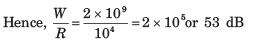QUESTION: 18

A slow FH binary FSK system with non coherent detection operates at ε0/J0 10, with hopping bandwidth of 2 GHz, and a bit rate of 10 kbps.

Que: If the jammer operates as a partial band jammar, the bandwidth occupancy for worst case jamming is

Solution:

The bandwidth of the worst partial-band jammer is α * W , where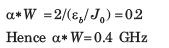QUESTION: 19

A slow FH binary FSK system with non coherent detection operates at ε0/J0 10, with hopping bandwidth of 2 GHz, and a bit rate of 10 kbps.

Que: The probability of error for the worst-case partial band jammer is

Solution:

The probability of error with worst-case partial-band jamming is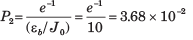QUESTION: 20

A slow FH binary FSK system with non coherent detection operates at ε0/J0 10, with hopping bandwidth of 2 GHz, and a bit rate of 10 kbps.

Que: The minimum hop rate for a FH spread spectrum system that will prevent a jammer from operating five onives away from the receiver is

Solution:

d = 5 miles = 8050 meters